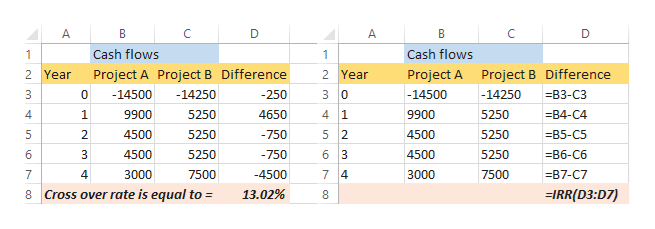# Part 1: The CFO of Cruz, inc. is considering Projects A and B which are considered equally risky; the projected cash flows for the projects are shown below.  T=01234Project A(14,500)9,9004,5004,5003000Project B (14,250)5,2505,2505,2507,5001. what is the "crossover rate" for the two projects? Show the difference between the two projects' timeline in the space above. round your answer to the nearest tenth of a percent 2. Provide the NPV's for both A and B at the rates in the table below. Round to the nearest dollar/whole number.  Interest Rate NPV project ANPV project B0%  6%  12%  18%  24%  3. Provide the IRR for each project in the table below. show each rate to the nearest tenth of a percent.Project A IRR? Project B IRR?4. Calculate the MIRR for project A, and B using a WACC (discount rate). use the "combination approach" by finding the future values(show your work). Show your new time line with amounts, then find the new IRR (or MIRR) using your calculator or exel. T=01234Project A(14,500)9,9004,5004,5003000Project B (14,250)5,2505,2505,2507,500

Question

Part 1: The CFO of Cruz, inc. is considering Projects A and B which are considered equally risky; the projected cash flows for the projects are shown below.

 T= 0 1 2 3 4 Project A (14,500) 9,900 4,500 4,500 3000 Project B (14,250) 5,250 5,250 5,250 7,500

1. what is the "crossover rate" for the two projects? Show the difference between the two projects' timeline in the space above. round your answer to the nearest tenth of a percent

2. Provide the NPV's for both A and B at the rates in the table below. Round to the nearest dollar/whole number.

 Interest Rate NPV project A NPV project B 0% 6% 12% 18% 24%

3. Provide the IRR for each project in the table below. show each rate to the nearest tenth of a percent.

Project A IRR?

Project B IRR?

4. Calculate the MIRR for project A, and B using a WACC (discount rate). use the "combination approach" by finding the future values(show your work). Show your new time line with amounts, then find the new IRR (or MIRR) using your calculator or exel.

 T= 0 1 2 3 4 Project A (14,500) 9,900 4,500 4,500 3000 Project B (14,250) 5,250 5,250 5,250 7,500
check_circle

Step 1

“Since you have posted a question with multiple sub-parts, we will solve first three sub-parts for you. To get remaining sub-part solved please repost the complete question and mention the sub-parts to be solved.”.

Step 2

Part 1:
Crossover rate refers to the cost of capital at which two projects will have same NPV. We have been given the cash flows, we can determine the cross over rate using excel.

Step 3

The value of crossover rate as calculated using exc...help_outlineImage TranscriptioncloseA D В Cash flows Cash flows 1 2 Year Project A Project B Difference 2 Year Project A Project B Difference -14250 3 0 -14500 0 -14500 -250 -14250 B3-C3 4 1 4 1 9900 5250 4650 9900 5250 -B4-С4 5 2 4500 2. 4500 5250 -750 5250 -B5-C5 6 3 4500 6 4500 5250 -750 5250 -В6-Сб 3000 -4500 7 4 7500 7 4 7500 3000 -B7-C7 Cross over rate is equal to 13.02% IRR(D3:D7) 8 co LO LO fullscreen

### Want to see the full answer?

See Solution

#### Want to see this answer and more?

Solutions are written by subject experts who are available 24/7. Questions are typically answered within 1 hour.*

See Solution
*Response times may vary by subject and question.
Tagged in